# Style Guide

### A set of standards, rules and conventions for the content of mathematical web pages.

This is a work in progress, and probably always will be. I am putting it together for my own benefit but realise that it may be of use to others too. Please let me know if you have any suggestions.

#### A Level

This qualification has capital initial letters and no hyphen.

#### Axes

The horizontal and vertical axes of a system of coordinates are written as x-axis and y-axis respectively (with hyphens).

#### Commas

Avoid using commas in large numbers. Use spaces, if necessary to separate groups of three digits. Fifty three million can be written as 53 000 000 rather than 53,000,000. In some countries a comma is used in place of a decimal point so avoiding commas avoids confusion.

#### Complement

Beware spelling this word with an i. Complement means something that completes or makes perfect as in complementary angles (sum to 180o). A compliment is a word, act or a circumstance, that expresses gratefulness, acknowledgement or honour to someone.

#### Diamond

A diamond shape should be referred to as a rhombus unless it has four right angles in which case it is a square (while still being a rhombus and a rectangle too!).

#### Difference

In mathematics (as distinct from its everyday meaning), difference means the numerical difference between two numbers. The difference between 10 and 3 is 10 − 3 = 7 however the difference between 3 and 10 is also 7. Difference is always positive as traditionally, the mathematical difference between two numbers refers to the distance on a number line between the points corresponding to each of the two numbers. One important exception is finding the common difference in an arithmetic sequence which could be negative.

#### Et cetera

The abbreviation is followed by a full stop etc.

#### Example

The abbreviation contains two full stops e.g.

#### Fewer

Use less with mass (continuous) nouns and fewer with count (discrete) nouns. Fewer pens, less clutter!

#### Figures

When you put a number at the beginning of a sentence it is recommended to write the number as a word (or words). If the number would be ridiculously long in words, you should rephrase the sentence so the number does not come at the beginning.

Use words for integers from one to ten inclusive, except: in percentages (e.g., 4%); and in sets of numerals, some of which are higher than ten.

#### Formulae

The plural of formula can be spelled either as formulas or, based on Latin, formulae. Transum will use the second spelling on the understanding that pupils will meet the first spelling in everyday life so this website can provide exposure to the alternative.

#### Fractions

When written as words, fractions should be hyphenated (one-half, three-quarters, etc.)

#### Hyphens

In addition to their use to join words to indicate that they have a combined meaning, hyphens can be used in place of the word to when used with figures i.e. 7-11, 5m–6m (not 5–6m)

#### Id est

That is to say: i.e.

#### Indices

Index, exponent and power are all words used to describe the small, superscript number in the expression 74 however power can also be used to describe the whole expression. The number seven is known as the base of the expression which can be referred to as seven to the power 4. Avoid saying seven to the fourth power as this could be misinterpreted as 7¼.

#### Intercepts/intersects

While lines may intersect, as soon as one of those lines is an axis the work becomes intercept. I'm not really sure why!

#### Learnt

Learnt is British English while learned is American English.

#### Million

The abbreviation for million is m though this is often confused with metres so should be avoided. A billion is a thousand million, a trillion a thousand billion, a quadrillion a thousand trillion.

#### Minus

The following definitions, including that of this controversial word, are taken away (pun) from the Oxford Dictionary.

• Subtract is a verb: If you subtract 4 from 7 you will be left with 3.
• Minus is defined as a preposition but can be used in two different ways: 7 minus 4 equals 3 but also the temperature was minus 2.
• Negative is an adjective: The temperature was negative 2.

#### Nought

Maths teachers are very good correcting their pupils when they use the letter O name when they really mean nought or zero. It is important to distinguish between letters (as used in algebra) and numbers. There are however exceptions to this rule that have come about by common usage:

1. James Bond's number is double 'O' seven
2. The first Scout camp took place in 1907 (nineteen 'O' seven)
3. My telephone number is 'O' two four nine seven one one one
4. A famous TV series was called Hawaii 5 'O'
5. It's my father's birthday. He's celebrating his big five 'O'.

#### Oblong

A rectangle with unequal adjacent sides. A rectangle with four equal sides is also known as a square.

#### Post Script

Post script should be in capitals with full stops as P.S.

#### Principle

Principal (with the 'al') is an adjective meaning foremost. It is also the name of a head of school and the initial investment involved in simple and compound interest calculations. Principle (with the 'le') is a noun with a similar meaning to idea or method as in Archimedes' Principle.

#### Prism

A solid figure whose two ends are equal parallel rectilinear figures and whose sides are parallelograms (If the lateral faces are rectangular and perpendicular to the bases, the prism is a right prism). A cylinder is not a prism.

#### Pupils

The young people sitting in front of teachers in schools are called pupils (rather than students) by the English National Curriculum documents.

#### Ruler

I remember many years ago some people saying that a ruler was a king or queen and the graduated stick used to measure line segments should be referred to as a rule. I was never very keen on that and now I have discovered that in the dictionary both rule and ruler have one of their many definitions similar to 'a straight usually graduated strip or cylinder of wood, metal etc., used to draw lines or measure distances'. Consequently I will happily continue to use the word ruler despite the fact that elsewhere on the web I find that rule is a ruler with graduations starting at its edge and a ruler's graduations begin a little way in from its edge.

#### Semicircle

Half a circle is one word, no hyphen between the i and c.

#### Treble vs Triple

Both words can be used as verbs (as well as adjectives) meaning to increase a value by a factor of three. Triple appears approximately three times for every instance of treble in British English (source Grammarist). In American English, treble is almost unheard of except in relation to sound and music.

#### Units

When used with ﬁgures, these lower-case abbreviations should follow immediately, with no space: 9am 3.30pm 17kg 388mm 108mph

#### Vocabulary for Arithmetic

• The total when numbers are added is called the sum
• minuend − subtrahend = difference
• multiplicand × multiplier = product
• dividend ÷ divisor = quotient

One term I hear occasionally but don't like is describing 6(2x-4) is six into two x minus four. I think the word 'into' implies division and 'lots of' would be more helpful.

I hear people describe factors as numbers which divide evenly into other numbers. I think exactly is better than evenly which suggests multiples of two.

The Transum website uses British English but here are some common American mathematical words that are different to the British English equivalent.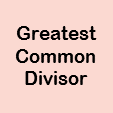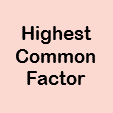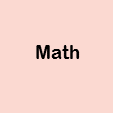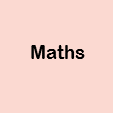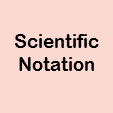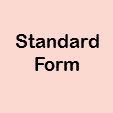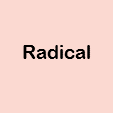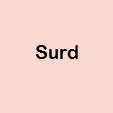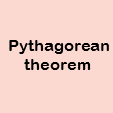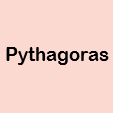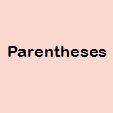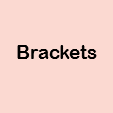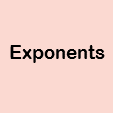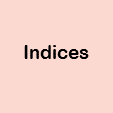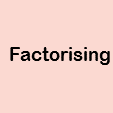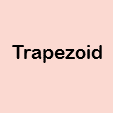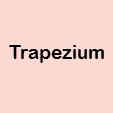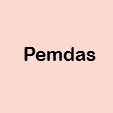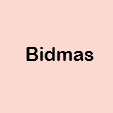If you are interested in the difference between British and American mathematical words there are some activities testing your knowledge here: Math vs Maths### Feedback

Do you have any comments? It is always useful to receive feedback and helps make this free resource even more useful for those learning Mathematics anywhere in the world. Click here to enter your comments.

The inspiration for this style guide came from the Grammar Girl podcast and the main references were the Oxford English Dictionary, The NCETM Mathematics Glossary and the Economist Style Guide.